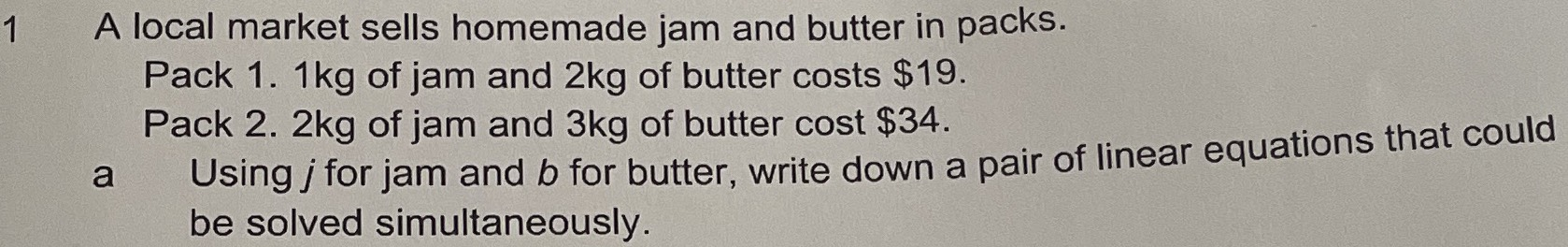### ¿Todavía tienes preguntas de matemáticas?

Pregunte a nuestros tutores expertos
Algebra
Pregunta$$1$$ A local market sells homemade jam and butter in packs. Pack 1. $$1 kg$$ of jam and $$2 kg$$ of butter costs $$\ 19$$ . Pack 2. $$2 kg$$ of jam and $$3 kg$$ of butter cost $$\ 34$$ . a Using $$j$$ for jam and $$b$$ for butter, write down a pair of linear equations that could be solved simultaneously.

$$j+ 2b= 19$$
$$2j+ 3b= 34$$ are the pair of linear equations